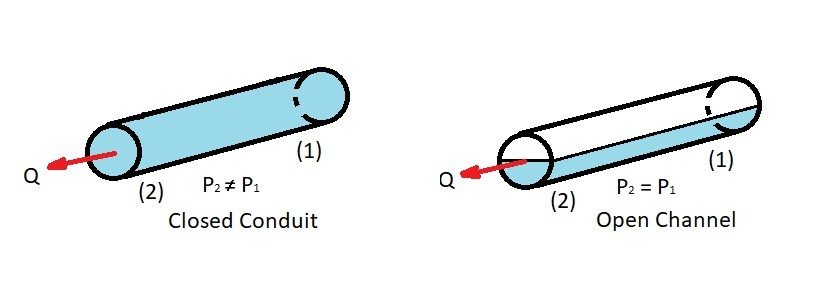# Pipe Flow

Generally, a fluid is transported from one point to another though a pipe.  As a result, knowing how to handle a pipe flow problem is important.  For this type of problem you will need to have an understanding of laminar and turbulent flow.  In addition, you will need to know when the flow becomes fully developed and what entrance length is required before this to occur.  Finally, you will need apply any viscous effects to the flow.  These aspects will be discussed in the following articles in this section.

### Pipe Flow: (Closed Conduit vs Open Channel)

Pipe flow is a branch of hydraulics.  It analyzes the flow through a closed conduit.  Generally, this conduit will have a circular cross-section, but not always.  When it does not have a circular cross-section you will need to find the hydraulic diameter so that you can treat it as a circular conduit.  To find the hydraulic diameter you will need to use the following equation.

(Eq 1) $D_H=\frac{4A}{P}$

$A$ = cross-sectional area

$P$ = wetted perimeter of the cross-section

Some typical examples of closed conduits that have a circular cross-section are water pipes and hydraulic hoses.  On the other hand an example of a non-circular cross-section is an air conditioning duct.

Finally, when analyzing a pipe flow problem the conduit must be completely filled with a fluid.  In turn if the conduit is not completely filled with one type of fluid it will need be treated as an open-channel conduit.  The reason why this is important is because the mechanism that drives the flow is different for open flow compared to closed flow.  Open flow is driven by gravity, while closed flow is driven by a pressure difference.  As a result, an open channel problem is not a pipe flow problem.Next

|